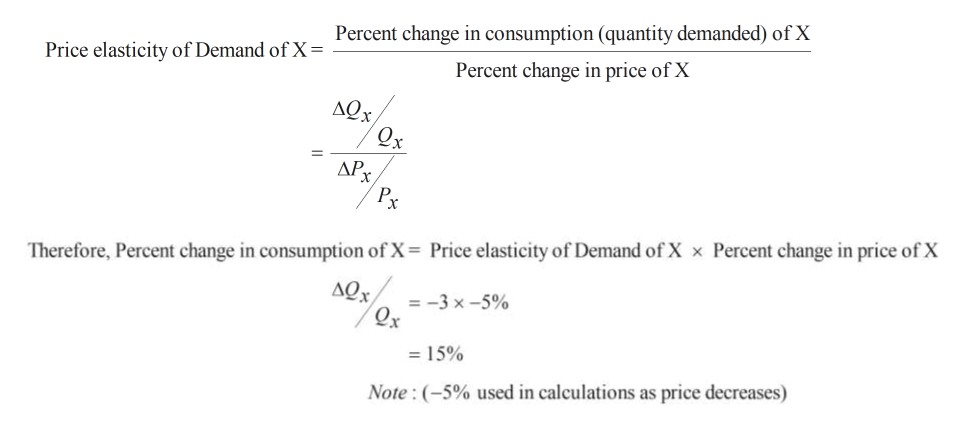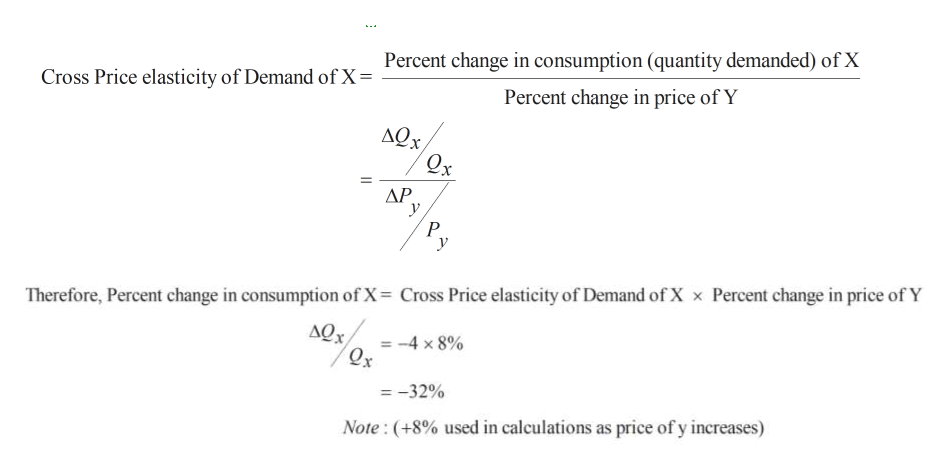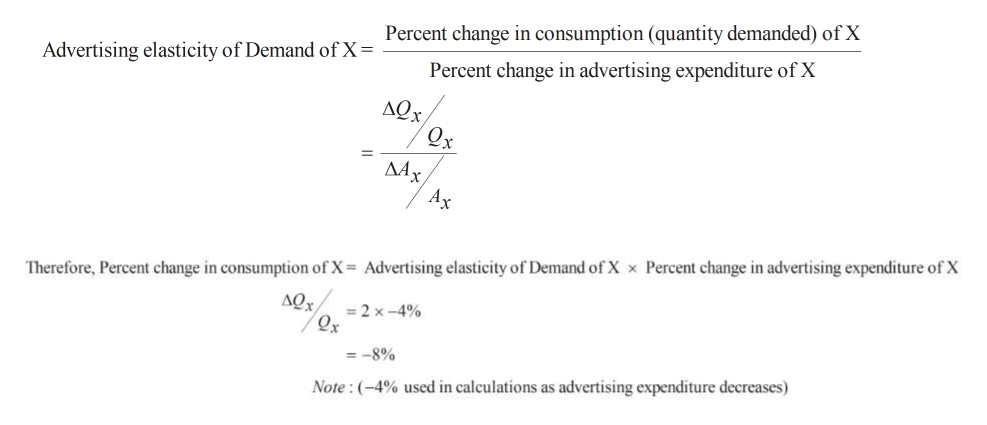# Suppose the own price elasticity of demand for good X is -3, its income elasticity is 1, its                 advertising elasticity is 2, and the cross-price elasticity of demand between it and good Y is -4.              Determine how much the consumption of good X will change if:The price of good X decreases by 5%The price of good Y increases by 8%Advertising decreases by 4%Income increases by 4%

Question

Suppose the own price elasticity of demand for good X is -3, its income elasticity is 1, its

advertising elasticity is 2, and the cross-price elasticity of demand between it and good Y is -4.
Determine how much the consumption of good X will change if:

1. The price of good X decreases by 5%
2. The price of good Y increases by 8%
4. Income increases by 4%
check_circleExpert Solution
Step 1

Part 1) When price of good X decreases by 5% , consumption of good X will increase by 15%help_outlineImage TranscriptionclosePrice elasticity of Demand of Y Percent change in consumption (quantity demanded) of X Percent change in price of X AQx Qx P Therefore, Percent change in consumption of X Price elasticity of Demand of X x Percent change in price of X AQx -3 x-5% Qx 15% Note: (-5% used in calculations as price decreases) fullscreen
Step 2

Part 2)

When price of Y increases by 8%,  consumption for X decreases by 32% as indicated by minus sign.

When price of Y increases by 8%, consumption of y decreases anyway but consumption of X also decreases as per our calculations. This suggests that X and Y are complement goods as their prices move together.

Change in consumption of X = -32%help_outlineImage TranscriptionclosePercent change in consumption (quantity demanded) of X Cross Price elasticity of Demand of X = Percent change in price of Y AQx Qx AP у P Therefore, Percent change in consumption of X= Cross Price elasticity of Demand of X x Percent change in price of Y =-4 x8% -32% Note: (+8% used in calculations as price of y increases) fullscreen
Step 3

Part 3)

Let Ax represent the units of advertising expenses.

Consumption of X decreases  by 8% when ad...help_outlineImage TranscriptioncloseAdvertising elasticity of Demand of X = rercent change in consumption (quantity demanded) of x Percent change in advertising expenditure of X дох, ДАх, Ax Advertising elasticity of Demand of X x Percent change in advertising expenditure of X Therefore, Percent change in consumption of X AQx 2 x-4% Qx =-8% Note:(-4% used in calculations as advertising expenditure decreases) fullscreen

### Want to see the full answer?

See Solution

#### Want to see this answer and more?

Solutions are written by subject experts who are available 24/7. Questions are typically answered within 1 hour*

See Solution
*Response times may vary by subject and question
Tagged in